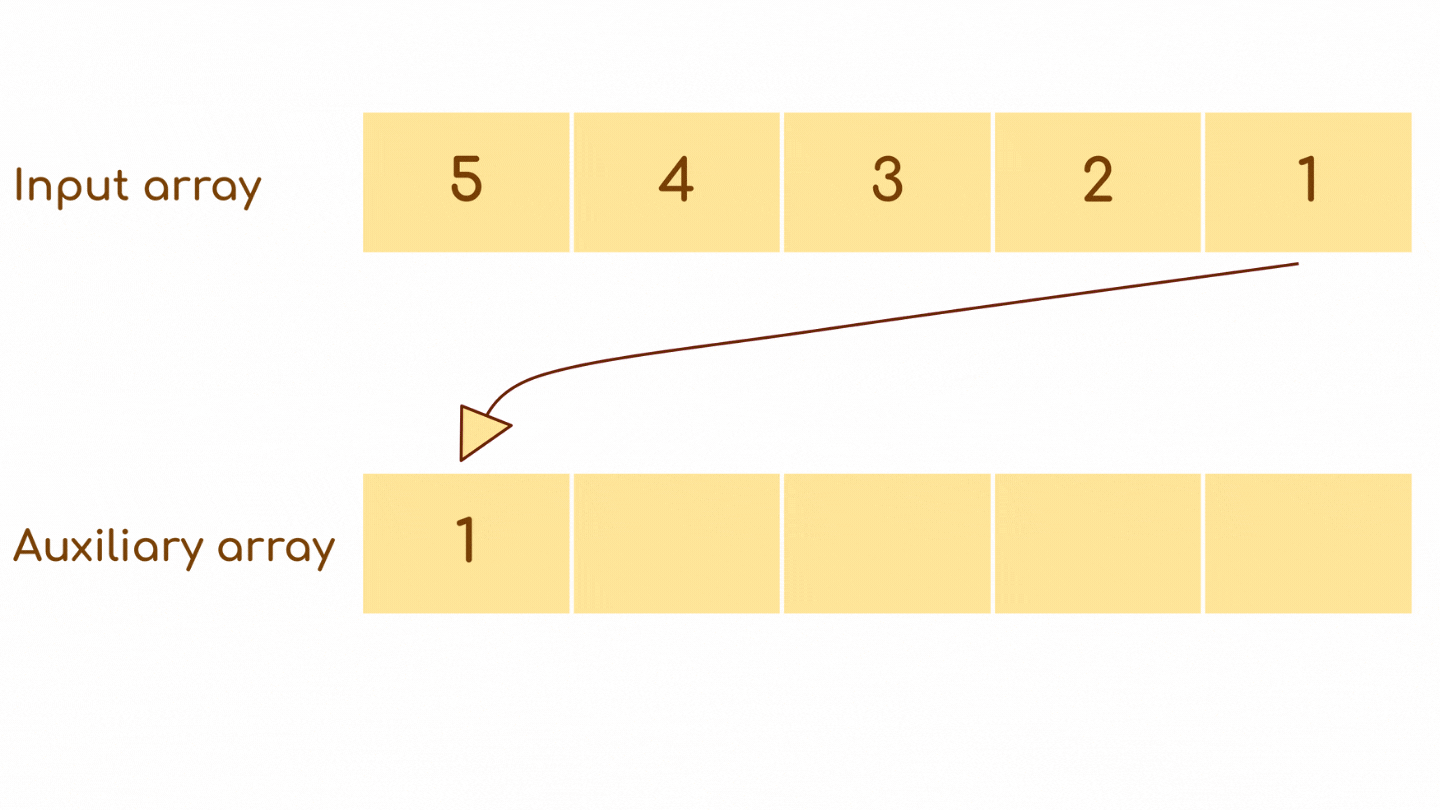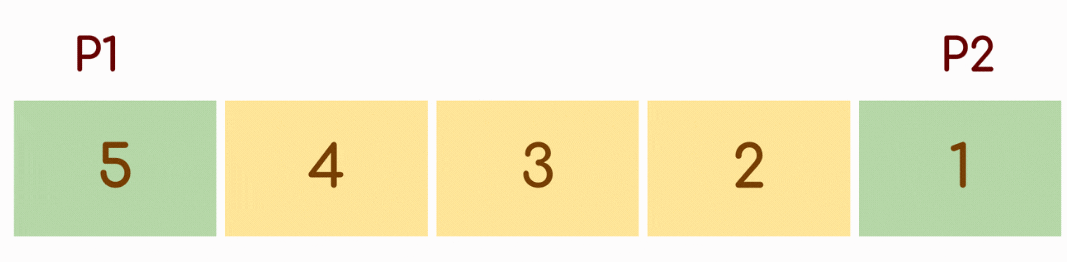# Reverse a given Array

Problem Statement: You are given an array. The task is to reverse the array and print it.

Examples:

```Example 1:
Input: N = 5, arr[] = {5,4,3,2,1}
Output: {1,2,3,4,5}
Explanation: Since the order of elements gets reversed the first element will occupy the fifth position, the second element occupies the fourth position and so on.

Example 2:
Input: N=6 arr[] = {10,20,30,40}
Output: {40,30,20,10}
Explanation: Since the order of elements gets reversed the first element will occupy the fifth position, the second element occupies the fourth position and so on.```

### Solution

Disclaimer: Don’t jump directly to the solution, try it out yourself first.

Solution 1: Using an extra array.

Approach: Declare an array,ans[] of the same size as the input array. Iterate from the back of the input array while storing the elements in ans[]  in opposite direction.Code:

## C++ Code

``````#include <iostream>
using namespace std;
//Function to print array
void printArray(int ans[], int n) {
cout << "The reversed array is:- " << endl;
for (int i = 0; i < n; i++) {
cout << ans[i] << " ";
}
}
//Function to reverse array using an auxiliary array
void reverseArray(int arr[], int n) {
int ans[n];
for (int i = n - 1; i >= 0; i--) {
ans[n - i - 1] = arr[i];
}
printArray(ans, n);
}
int main() {
int n = 5;
int arr[] = {5,4,3,2,1};
reverseArray(arr, n);
return 0;
}
``````

Output:

The reversed array is:-
1 2 3 4 5

Time Complexity: O(n), single-pass for reversing array.

Space Complexity: O(n), for the extra array used.

## Java Code

``````public class Main {
//Function to print array
static void printArray(int ans[], int n) {
System.out.print("Reversed array is:- \n");
for (int i = 0; i < n; i++) {
System.out.print(ans[i] + " ");
}
}
//Function to reverse array using an auxiliary array
static void reverseArray(int arr[], int n) {
int[] ans = new int[n];
for (int i = n - 1; i >= 0; i--) {
ans[n - i - 1] = arr[i];
}
printArray(ans, n);
}
public static void main(String[] args) {
int n = 5;
int arr[] = { 5, 4, 3, 2, 1};
reverseArray(arr, n);
}
}
``````

Output:

The reversed array is:-
1 2 3 4 5

Time Complexity: O(n), single-pass for reversing array.

Space Complexity: O(n), for the extra array used.

Solution 2:  Space optimized iterative method

Approach: Unlike the previous method we use the same array to obtain the result. Follow the steps below.

• Keep a pointer p1  at the first index and another p2 at the last index of array.
• Swap the elements  pointed by p1 and p2,Post swapping increment p1 and decrement p2.
• This process is repeated for only the first n/2 elements where n is the length of array.

Note: Swapping all the n elements instead of n/2 elements leaves the array unaltered.Code:

## C++ Code

``````#include <iostream>

using namespace std;
//Function to print array
void printArray(int arr[], int n) {
cout << "The reversed array is:- " << endl;
for (int i = 0; i < n; i++) {
cout << arr[i] << " ";
}
}
//Function to reverse array
void reverseArray(int arr[], int n) {
int p1 = 0, p2 = n - 1;
while (p1 < p2) {
swap(arr[p1], arr[p2]);
p1++; p2--;
}
printArray(arr, n);
}
int main() {
int n = 5;
int arr[] = { 5, 4, 3, 2, 1};
reverseArray(arr, n);
return 0;
}
``````

Output:

The reversed array is:-
1 2 3 4 5

Time Complexity: O(n), single-pass involved.

Space Complexity: O(1)

## Java Code

``````public class Main {
//Function to print array
static void printArray(int arr[], int n) {
System.out.print("Reversed array is:- \n");
for (int i = 0; i < n; i++) {
System.out.print(arr[i] + " ");
}
}
//Function to reverse array
static void reverseArray(int arr[], int n) {
int p1 = 0, p2 = n - 1;
while (p1 < p2) {
int tmp = arr[p1];
arr[p1] = arr[p2];
arr[p2] = tmp;
p1++;
p2--;
}
printArray(arr, n);
}
public static void main(String[] args) {
int n = 5;
int arr[] = { 5, 4, 3, 2, 1};
reverseArray(arr, n);

}
}
``````

Output:

The reversed array is:-
1 2 3 4 5

Time Complexity: O(n), single-pass involved.

Space Complexity: O(1)

Solution 3: Recursive method

Approach: The recursive method has an approach almost similar to the iterative one. The approach has been broken down into some steps for simplicity.

1. Create a function that takes an array ,start index and end index of the array as parameters.
2. Swap the elements present  at start and end index,
3. The portion of array left to be reversed is arr[start+1,end-1]. Make a recursive call to reverse the rest of array. While calling recursion pass start +1  and end – 1 as parameters for the shrinked array. Repeat step 2.
4. Continue recursion as long as ‘start < end’ condition is satisfied. This is the base case for our recursion.

Code:

## C++ Code

``````#include <iostream>

using namespace std;
//Function to print array
void printArray(int arr[], int n) {
cout << "The reversed array is:- " << endl;
for (int i = 0; i < n; i++) {
cout << arr[i] << " ";
}
}
//Function to reverse array using recursion
void reverseArray(int arr[], int start, int end) {
if (start < end) {
swap(arr[start], arr[end]);
reverseArray(arr, start + 1, end - 1);
}
}
int main() {
int n = 5;
int arr[] = { 5, 4, 3, 2, 1 };
reverseArray(arr, 0, n - 1);
printArray(arr, n);
return 0;
}
``````

Output:

The reversed array is:-
1 2 3 4 5

Time Complexity: O(n)

Space Complexity: O(1)

## Java Code

``````public class Main {
//Function to print array
static void printArray(int arr[], int n) {
System.out.print("Reversed array is:- \n");
for (int i = 0; i < n; i++) {
System.out.print(arr[i] + " ");
}
}
//Function to reverse array using recursion
static void reverseArray(int arr[], int start, int end) {
if (start < end) {
int tmp = arr[start];
arr[start] = arr[end];
arr[end] = tmp;
reverseArray(arr, start + 1, end - 1);
}
}
public static void main(String[] args) {
int n = 5;
int arr[] = { 5, 4, 3, 2, 1};
reverseArray(arr, 0, n - 1);
printArray(arr, n);
}
}

``````

Output:

The reversed array is:-
1 2 3 4 5

Time Complexity: O(n)

Space Complexity: O(1)

Solution 4: Using library function (New Approach)

Approach: C++ and Java have inbuilt functions to reverse an array.

For C++:-

The std::reverse function in C++ is predefined in a header file algorithm.

Syntax: array_name.reverse(BidirectionalIterator first, BidirectionalIterator last)

Bidirectional iterators first and last indicate the starting and ending positions in the array. Elements that lie in this range are reversed. Note that this range includes the first but excludes the last element.

For Java:-

The reverse method in java can be imported from the Collections class present in java.util package.

Note:

1. Since this method expects an object as a parameter we have to convert the array into a list object using asList().
2. Java collections require wrapper classes instead of primitive data type.In order to  reverse an integer array use Integer instead of int.

Syntax: Collections.reverse(class_obj);

Code:

## C++ Code

``````#include <iostream>
#include<algorithm>

using namespace std;
//Function to print array
void printArray(int arr[], int n) {
cout << "The reversed array is:- " << endl;
for (int i = 0; i < n; i++) {
cout << arr[i] << " ";
}
}
//Reverse array using library function
void reverseArray(int arr[], int n) {
//Reversing elements from index 0 to n-1
reverse(arr, arr + n);
}
int main() {
int n = 5;
int arr[] = { 5, 4, 3, 2, 1 };
reverseArray(arr, n);
printArray(arr, n);
return 0;
}
``````

Output:

The reversed array is:-
1 2 3 4 5

Time Complexity: O(n)

Space Complexity: O(1)

## Java Code

``````import java.util.*;
public class Main {
//Function to print array
static void printArray(Integer arr[], int n) {
System.out.print("Reversed array is:- \n");
for (int i = 0; i < n; i++) {
System.out.print(arr[i] + " ");
}
}
//Reverse array using library function
static void reverseArray(Integer arr[]) {
//fetching array as list object
//reversing the fetched object
Collections.reverse(Arrays.asList(arr));
}
public static void main(String[] args) {
int n = 5;
Integer arr[] = {5,4,3,2,1};
reverseArray(arr);
printArray(arr, n);
}
}
``````

Output:

The reversed array is:-
1 2 3 4 5

Time Complexity: O(n)

Space Complexity: O(1)

Special thanks to Somparna Chakrabarti for contributing to this article on takeUforward. If you also wish to share your knowledge with the takeUforward fam, please check out this article